# Problem of the Day #7 March 31, 2020

Mar 31, 2020Problem of the Day #7 March 31, 2020

Grade 6: Solve the following equations: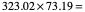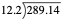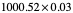Grade 7: Solve the following equations:

-47 + 23 =                  15 - ( -7 ) =             22 + ( -12) =        -52 - ( - 26) =

Grade 8: Solve the following inequalities: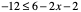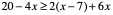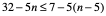Algebra: Write the equation for the line with the given characteristics:

parallel to 2x+3y = 5 containing the point (6, -5)

perpendicular to -4x+2y = 6  with a y-intercept of 3

containing the points ( 3, -7 ) and ( 8, -12)

Geometry: Find the area of the following polygons:Algebra 2: Solve the following equations: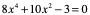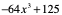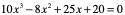Trigonometry: Solve the following triangle. Be sure to find the length of each side and the measure of each anglePre-Calculus: Solve the following expressions.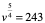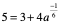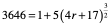Grade 7: -24, 22, 10, -26

Grade 8: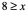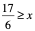all real numbers

Algebra: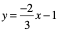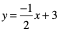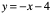Geometry:  60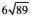Algebra 2: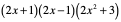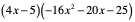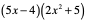Trigonometry: side b = 45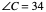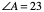Pre-Calculus:    81, 64, 16